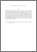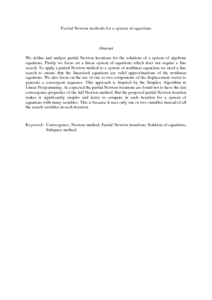# Partial Newton methods for a system of equations

## Citation

Goh, Bean San and Leong, Wah June and Siri, Zailan (2013) Partial Newton methods for a system of equations. Numerical Algebra, Control and Optimization, 3 (3). pp. 463-469. ISSN 2155-3289; ESSN: 2155-3297

## Abstract

We define and analyse partial Newton iterations for the solutions of a system of algebraic equations. Firstly we focus on a linear system of equations which does not require a line search. To apply a partial Newton method to a system of nonlinear equations we need a line search to ensure that the linearized equations are valid approximations of the nonlinear equations. We also focus on the use of one or two components of the displacement vector to generate a convergent sequence. This approach is inspired by the Simplex Algorithm in Linear Programming. As expected the partial Newton iterations are found not to have the fast convergence properties of the full Newton method. But the proposed partial Newton iteration makes it significantly simpler and faster to compute in each iteration for a system of equations with many variables. This is because it uses only one or two variables instead of all the search variables in each iteration.Preview
PDF (Abstract)
Partial Newton methods for a system of equations.pdfView Item